Shortcuts

# torchaudio.transforms¶

Transforms are common audio transforms. They can be chained together using torch.nn.Sequential

## Utility¶

### AmplitudeToDB¶

class torchaudio.transforms.AmplitudeToDB(stype: str = 'power', top_db: Optional[float] = None)[source]

Turn a tensor from the power/amplitude scale to the decibel scale.

This output depends on the maximum value in the input tensor, and so may return different values for an audio clip split into snippets vs. a a full clip.

Parameters
• stype (str, optional) – scale of input tensor ('power' or 'magnitude'). The power being the elementwise square of the magnitude. (Default: 'power')

• top_db (float or None, optional) – minimum negative cut-off in decibels. A reasonable number is 80. (Default: None)

forward(x: torch.Tensor)torch.Tensor[source]

Numerically stable implementation from Librosa.

https://librosa.org/doc/latest/generated/librosa.amplitude_to_db.html

Parameters

x (Tensor) – Input tensor before being converted to decibel scale.

Returns

Output tensor in decibel scale.

Return type

Tensor

### MelScale¶

class torchaudio.transforms.MelScale(n_mels: int = 128, sample_rate: int = 16000, f_min: float = 0.0, f_max: Optional[float] = None, n_stft: int = 201, norm: Optional[str] = None, mel_scale: str = 'htk')[source]

Turn a normal STFT into a mel frequency STFT, using a conversion matrix. This uses triangular filter banks.

Parameters
• n_mels (int, optional) – Number of mel filterbanks. (Default: 128)

• sample_rate (int, optional) – Sample rate of audio signal. (Default: 16000)

• f_min (float, optional) – Minimum frequency. (Default: 0.)

• f_max (float or None, optional) – Maximum frequency. (Default: sample_rate // 2)

• n_stft (int, optional) – Number of bins in STFT. See n_fft in Spectrogram. (Default: 201)

• norm (str or None, optional) – If 'slaney', divide the triangular mel weights by the width of the mel band (area normalization). (Default: None)

• mel_scale (str, optional) – Scale to use: htk or slaney. (Default: htk)

torchaudio.functional.melscale_fbanks() - The function used to generate the filter banks.

forward(specgram: torch.Tensor)torch.Tensor[source]
Parameters

specgram (Tensor) – A spectrogram STFT of dimension (…, freq, time).

Returns

Mel frequency spectrogram of size (…, n_mels, time).

Return type

Tensor

### InverseMelScale¶

class torchaudio.transforms.InverseMelScale(n_stft: int, n_mels: int = 128, sample_rate: int = 16000, f_min: float = 0.0, f_max: Optional[float] = None, max_iter: int = 100000, tolerance_loss: float = 1e-05, tolerance_change: float = 1e-08, sgdargs: Optional[dict] = None, norm: Optional[str] = None, mel_scale: str = 'htk')[source]

Solve for a normal STFT from a mel frequency STFT, using a conversion matrix. This uses triangular filter banks.

It minimizes the euclidian norm between the input mel-spectrogram and the product between the estimated spectrogram and the filter banks using SGD.

Parameters
• n_stft (int) – Number of bins in STFT. See n_fft in Spectrogram.

• n_mels (int, optional) – Number of mel filterbanks. (Default: 128)

• sample_rate (int, optional) – Sample rate of audio signal. (Default: 16000)

• f_min (float, optional) – Minimum frequency. (Default: 0.)

• f_max (float or None, optional) – Maximum frequency. (Default: sample_rate // 2)

• max_iter (int, optional) – Maximum number of optimization iterations. (Default: 100000)

• tolerance_loss (float, optional) – Value of loss to stop optimization at. (Default: 1e-5)

• tolerance_change (float, optional) – Difference in losses to stop optimization at. (Default: 1e-8)

• sgdargs (dict or None, optional) – Arguments for the SGD optimizer. (Default: None)

• norm (str or None, optional) – If ‘slaney’, divide the triangular mel weights by the width of the mel band (area normalization). (Default: None)

• mel_scale (str, optional) – Scale to use: htk or slaney. (Default: htk)

forward(melspec: torch.Tensor)torch.Tensor[source]
Parameters

melspec (Tensor) – A Mel frequency spectrogram of dimension (…, n_mels, time)

Returns

Linear scale spectrogram of size (…, freq, time)

Return type

Tensor

### MuLawEncoding¶

class torchaudio.transforms.MuLawEncoding(quantization_channels: int = 256)[source]

Encode signal based on mu-law companding. For more info see the Wikipedia Entry

This algorithm assumes the signal has been scaled to between -1 and 1 and returns a signal encoded with values from 0 to quantization_channels - 1

Parameters

quantization_channels (int, optional) – Number of channels. (Default: 256)

Example
>>> waveform, sample_rate = torchaudio.load('test.wav', normalize=True)
>>> transform = torchaudio.transforms.MuLawEncoding(quantization_channels=512)
>>> mulawtrans = transform(waveform)

forward(x: torch.Tensor)torch.Tensor[source]
Parameters

x (Tensor) – A signal to be encoded.

Returns

An encoded signal.

Return type

Tensor

### MuLawDecoding¶

class torchaudio.transforms.MuLawDecoding(quantization_channels: int = 256)[source]

Decode mu-law encoded signal. For more info see the Wikipedia Entry

This expects an input with values between 0 and quantization_channels - 1 and returns a signal scaled between -1 and 1.

Parameters

quantization_channels (int, optional) – Number of channels. (Default: 256)

Example
>>> waveform, sample_rate = torchaudio.load('test.wav', normalize=True)
>>> transform = torchaudio.transforms.MuLawDecoding(quantization_channels=512)
>>> mulawtrans = transform(waveform)

forward(x_mu: torch.Tensor)torch.Tensor[source]
Parameters

x_mu (Tensor) – A mu-law encoded signal which needs to be decoded.

Returns

The signal decoded.

Return type

Tensor

### Resample¶

class torchaudio.transforms.Resample(orig_freq: int = 16000, new_freq: int = 16000, resampling_method: str = 'sinc_interpolation', lowpass_filter_width: int = 6, rolloff: float = 0.99, beta: Optional[float] = None, *, dtype: Optional[torch.dtype] = None)[source]

Resample a signal from one frequency to another. A resampling method can be given.

Note

If resampling on waveforms of higher precision than float32, there may be a small loss of precision because the kernel is cached once as float32. If high precision resampling is important for your application, the functional form will retain higher precision, but run slower because it does not cache the kernel. Alternatively, you could rewrite a transform that caches a higher precision kernel.

Parameters
• orig_freq (int, optional) – The original frequency of the signal. (Default: 16000)

• new_freq (int, optional) – The desired frequency. (Default: 16000)

• resampling_method (str, optional) – The resampling method to use. Options: [sinc_interpolation, kaiser_window] (Default: 'sinc_interpolation')

• lowpass_filter_width (int, optional) – Controls the sharpness of the filter, more == sharper but less efficient. (Default: 6)

• rolloff (float, optional) – The roll-off frequency of the filter, as a fraction of the Nyquist. Lower values reduce anti-aliasing, but also reduce some of the highest frequencies. (Default: 0.99)

• beta (float or None, optional) – The shape parameter used for kaiser window.

• dtype (torch.device, optional) – Determnines the precision that resampling kernel is pre-computed and cached. If not provided, kernel is computed with torch.float64 then cached as torch.float32. If you need higher precision, provide torch.float64, and the pre-computed kernel is computed and cached as torch.float64. If you use resample with lower precision, then instead of providing this providing this argument, please use Resample.to(dtype), so that the kernel generation is still carried out on torch.float64.

Example
>>> waveform, sample_rate = torchaudio.load('test.wav', normalize=True)
>>> transform = transforms.Resample(sample_rate, sample_rate/10)
>>> waveform = transform(waveform)

forward(waveform: torch.Tensor)torch.Tensor[source]
Parameters

waveform (Tensor) – Tensor of audio of dimension (…, time).

Returns

Output signal of dimension (…, time).

Return type

Tensor

class torchaudio.transforms.FrequencyMasking(freq_mask_param: int, iid_masks: bool = False)[source]

Apply masking to a spectrogram in the frequency domain.

Proposed in SpecAugment .

Parameters
• freq_mask_param (int) – maximum possible length of the mask. Indices uniformly sampled from [0, freq_mask_param).

• iid_masks (bool, optional) – whether to apply different masks to each example/channel in the batch. (Default: False) This option is applicable only when the input tensor is 4D.

Example
>>> spectrogram = torchaudio.transforms.Spectrogram()
>>>
>>> original = spectrogram(waveform)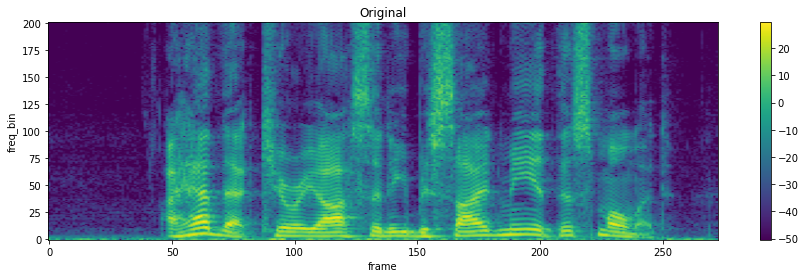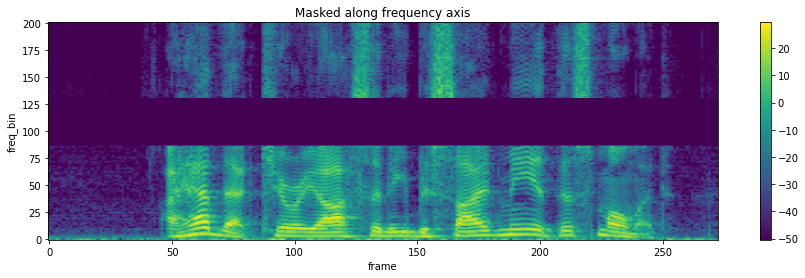forward(specgram: torch.Tensor, mask_value: float = 0.0)torch.Tensor
Parameters
• specgram (Tensor) – Tensor of dimension (…, freq, time).

• mask_value (float) – Value to assign to the masked columns.

Returns

Masked spectrogram of dimensions (…, freq, time).

Return type

Tensor

class torchaudio.transforms.TimeMasking(time_mask_param: int, iid_masks: bool = False)[source]

Apply masking to a spectrogram in the time domain.

Proposed in SpecAugment .

Parameters
• time_mask_param (int) – maximum possible length of the mask. Indices uniformly sampled from [0, time_mask_param).

• iid_masks (bool, optional) – whether to apply different masks to each example/channel in the batch. (Default: False) This option is applicable only when the input tensor is 4D.

Example
>>> spectrogram = torchaudio.transforms.Spectrogram()
>>>
>>> original = spectrogram(waveform)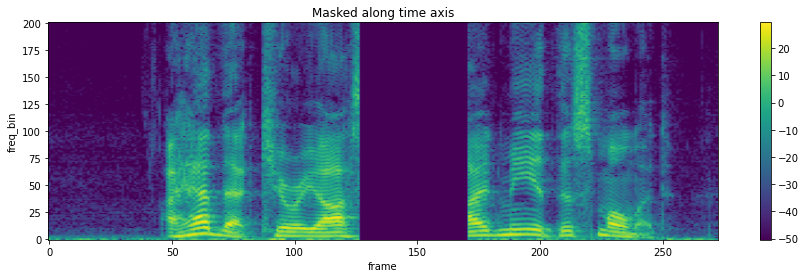forward(specgram: torch.Tensor, mask_value: float = 0.0)torch.Tensor
Parameters
• specgram (Tensor) – Tensor of dimension (…, freq, time).

• mask_value (float) – Value to assign to the masked columns.

Returns

Masked spectrogram of dimensions (…, freq, time).

Return type

Tensor

### TimeStretch¶

class torchaudio.transforms.TimeStretch(hop_length: Optional[int] = None, n_freq: int = 201, fixed_rate: Optional[float] = None)[source]

Stretch stft in time without modifying pitch for a given rate.

Proposed in SpecAugment .

Parameters
• hop_length (int or None, optional) – Length of hop between STFT windows. (Default: win_length // 2)

• n_freq (int, optional) – number of filter banks from stft. (Default: 201)

• fixed_rate (float or None, optional) – rate to speed up or slow down by. If None is provided, rate must be passed to the forward method. (Default: None)

Example
>>> spectrogram = torchaudio.transforms.Spectrogram()
>>> stretch = torchaudio.transforms.TimeStretch()
>>>
>>> original = spectrogram(waveform)
>>> streched_1_2 = stretch(original, 1.2)
>>> streched_0_9 = stretch(original, 0.9)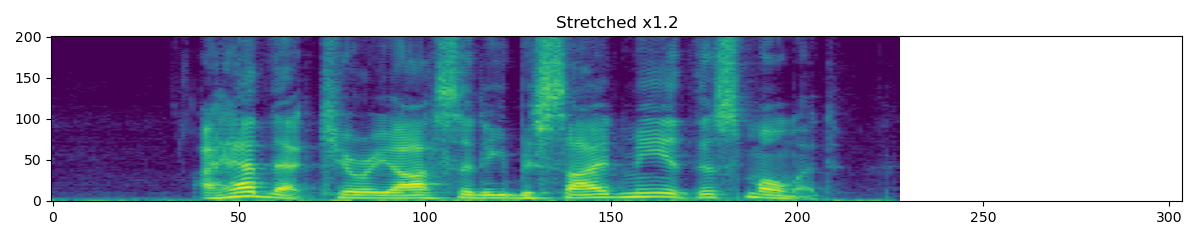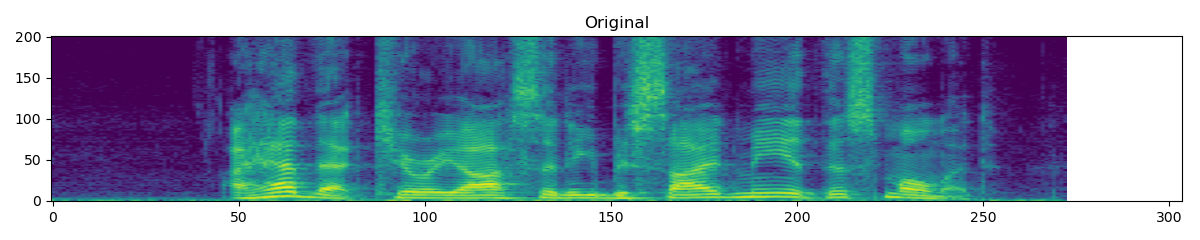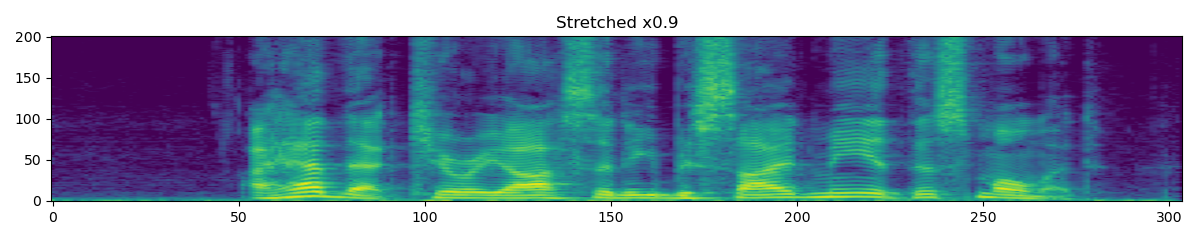forward(complex_specgrams: torch.Tensor, overriding_rate: Optional[float] = None)torch.Tensor[source]
Parameters
• complex_specgrams (Tensor) – Either a real tensor of dimension of (…, freq, num_frame, complex=2) or a tensor of dimension (…, freq, num_frame) with complex dtype.

• overriding_rate (float or None, optional) – speed up to apply to this batch. If no rate is passed, use self.fixed_rate. (Default: None)

Returns

Stretched spectrogram. The resulting tensor is of the same dtype as the input spectrogram, but the number of frames is changed to ceil(num_frame / rate).

Return type

Tensor

class torchaudio.transforms.Fade(fade_in_len: int = 0, fade_out_len: int = 0, fade_shape: str = 'linear')[source]

Add a fade in and/or fade out to an waveform.

Parameters
• fade_in_len (int, optional) – Length of fade-in (time frames). (Default: 0)

• fade_out_len (int, optional) – Length of fade-out (time frames). (Default: 0)

• fade_shape (str, optional) – Shape of fade. Must be one of: “quarter_sine”, "half_sine", "linear", "logarithmic", "exponential". (Default: "linear")

Example
>>> waveform, sample_rate = torchaudio.load('test.wav', normalize=True)
>>> faded_waveform = transform(waveform)

forward(waveform: torch.Tensor)torch.Tensor[source]
Parameters

waveform (Tensor) – Tensor of audio of dimension (…, time).

Returns

Tensor of audio of dimension (…, time).

Return type

Tensor

### Vol¶

class torchaudio.transforms.Vol(gain: float, gain_type: str = 'amplitude')[source]

Add a volume to an waveform.

Parameters
• gain (float) – Interpreted according to the given gain_type: If gain_type = amplitude, gain is a positive amplitude ratio. If gain_type = power, gain is a power (voltage squared). If gain_type = db, gain is in decibels.

• gain_type (str, optional) – Type of gain. One of: amplitude, power, db (Default: amplitude)

forward(waveform: torch.Tensor)torch.Tensor[source]
Parameters

waveform (Tensor) – Tensor of audio of dimension (…, time).

Returns

Tensor of audio of dimension (…, time).

Return type

Tensor

## Complex Utility¶

### ComplexNorm¶

class torchaudio.transforms.ComplexNorm(power: float = 1.0)[source]

Compute the norm of complex tensor input.

Parameters

power (float, optional) – Power of the norm. (Default: to 1.0)

Example
>>> complex_tensor = ... #  Tensor shape of (…, complex=2)
>>> transform = transforms.ComplexNorm(power=2)
>>> complex_norm = transform(complex_tensor)

forward(complex_tensor: torch.Tensor)torch.Tensor[source]
Parameters

complex_tensor (Tensor) – Tensor shape of (…, complex=2).

Returns

norm of the input tensor, shape of (…, ).

Return type

Tensor

## Feature Extractions¶

### Spectrogram¶

class torchaudio.transforms.Spectrogram(n_fft: int = 400, win_length: Optional[int] = None, hop_length: Optional[int] = None, pad: int = 0, window_fn: Callable[[...], torch.Tensor] = <built-in method hann_window of type object>, power: Optional[float] = 2.0, normalized: bool = False, wkwargs: Optional[dict] = None, center: bool = True, pad_mode: str = 'reflect', onesided: bool = True, return_complex: bool = True)[source]

Create a spectrogram from a audio signal.

Parameters
• n_fft (int, optional) – Size of FFT, creates n_fft // 2 + 1 bins. (Default: 400)

• win_length (int or None, optional) – Window size. (Default: n_fft)

• hop_length (int or None, optional) – Length of hop between STFT windows. (Default: win_length // 2)

• pad (int, optional) – Two sided padding of signal. (Default: 0)

• window_fn (Callable[.., Tensor], optional) – A function to create a window tensor that is applied/multiplied to each frame/window. (Default: torch.hann_window)

• power (float or None, optional) – Exponent for the magnitude spectrogram, (must be > 0) e.g., 1 for energy, 2 for power, etc. If None, then the complex spectrum is returned instead. (Default: 2)

• normalized (bool, optional) – Whether to normalize by magnitude after stft. (Default: False)

• wkwargs (dict or None, optional) – Arguments for window function. (Default: None)

• center (bool, optional) – whether to pad waveform on both sides so that the $$t$$-th frame is centered at time $$t \times \text{hop\_length}$$. (Default: True)

• pad_mode (string, optional) – controls the padding method used when center is True. (Default: "reflect")

• onesided (bool, optional) – controls whether to return half of results to avoid redundancy (Default: True)

• return_complex (bool, optional) – Indicates whether the resulting complex-valued Tensor should be represented with native complex dtype, such as torch.cfloat and torch.cdouble, or real dtype mimicking complex value with an extra dimension for real and imaginary parts. (See also torch.view_as_real.) This argument is only effective when power=None. It is ignored for cases where power is a number as in those cases, the returned tensor is power spectrogram, which is a real-valued tensor.

Example
>>> waveform, sample_rate = torchaudio.load('test.wav', normalize=True)
>>> transform = torchaudio.transforms.Spectrogram(n_fft=800)
>>> spectrogram = transform(waveform)

forward(waveform: torch.Tensor)torch.Tensor[source]
Parameters

waveform (Tensor) – Tensor of audio of dimension (…, time).

Returns

Dimension (…, freq, time), where freq is n_fft // 2 + 1 where n_fft is the number of Fourier bins, and time is the number of window hops (n_frame).

Return type

Tensor

### InverseSpectrogram¶

class torchaudio.transforms.InverseSpectrogram(n_fft: int = 400, win_length: Optional[int] = None, hop_length: Optional[int] = None, pad: int = 0, window_fn: Callable[[...], torch.Tensor] = <built-in method hann_window of type object>, normalized: bool = False, wkwargs: Optional[dict] = None, center: bool = True, pad_mode: str = 'reflect', onesided: bool = True)[source]

Create an inverse spectrogram to recover an audio signal from a spectrogram.

Parameters
• n_fft (int, optional) – Size of FFT, creates n_fft // 2 + 1 bins. (Default: 400)

• win_length (int or None, optional) – Window size. (Default: n_fft)

• hop_length (int or None, optional) – Length of hop between STFT windows. (Default: win_length // 2)

• pad (int, optional) – Two sided padding of signal. (Default: 0)

• window_fn (Callable[.., Tensor], optional) – A function to create a window tensor that is applied/multiplied to each frame/window. (Default: torch.hann_window)

• normalized (bool, optional) – Whether the spectrogram was normalized by magnitude after stft. (Default: False)

• wkwargs (dict or None, optional) – Arguments for window function. (Default: None)

• center (bool, optional) – whether the signal in spectrogram was padded on both sides so that the $$t$$-th frame is centered at time $$t \times \text{hop\_length}$$. (Default: True)

• pad_mode (string, optional) – controls the padding method used when center is True. (Default: "reflect")

• onesided (bool, optional) – controls whether spectrogram was used to return half of results to avoid redundancy (Default: True)

Example
>>> batch, freq, time = 2, 257, 100
>>> length = 25344
>>> spectrogram = torch.randn(batch, freq, time, dtype=torch.cdouble)
>>> transform = transforms.InverseSpectrogram(n_fft=512)
>>> waveform = transform(spectrogram, length)

forward(spectrogram: torch.Tensor, length: Optional[int] = None)torch.Tensor[source]
Parameters
• spectrogram (Tensor) – Complex tensor of audio of dimension (…, freq, time).

• length (int or None, optional) – The output length of the waveform.

Returns

Dimension (…, time), Least squares estimation of the original signal.

Return type

Tensor

### MelSpectrogram¶

class torchaudio.transforms.MelSpectrogram(sample_rate: int = 16000, n_fft: int = 400, win_length: Optional[int] = None, hop_length: Optional[int] = None, f_min: float = 0.0, f_max: Optional[float] = None, pad: int = 0, n_mels: int = 128, window_fn: Callable[[...], torch.Tensor] = <built-in method hann_window of type object>, power: float = 2.0, normalized: bool = False, wkwargs: Optional[dict] = None, center: bool = True, pad_mode: str = 'reflect', onesided: bool = True, norm: Optional[str] = None, mel_scale: str = 'htk')[source]

Create MelSpectrogram for a raw audio signal.

This is a composition of torchaudio.transforms.Spectrogram() and and torchaudio.transforms.MelScale().

Sources
Parameters
• sample_rate (int, optional) – Sample rate of audio signal. (Default: 16000)

• n_fft (int, optional) – Size of FFT, creates n_fft // 2 + 1 bins. (Default: 400)

• win_length (int or None, optional) – Window size. (Default: n_fft)

• hop_length (int or None, optional) – Length of hop between STFT windows. (Default: win_length // 2)

• f_min (float, optional) – Minimum frequency. (Default: 0.)

• f_max (float or None, optional) – Maximum frequency. (Default: None)

• pad (int, optional) – Two sided padding of signal. (Default: 0)

• n_mels (int, optional) – Number of mel filterbanks. (Default: 128)

• window_fn (Callable[.., Tensor], optional) – A function to create a window tensor that is applied/multiplied to each frame/window. (Default: torch.hann_window)

• power (float, optional) – Exponent for the magnitude spectrogram, (must be > 0) e.g., 1 for energy, 2 for power, etc. (Default: 2)

• normalized (bool, optional) – Whether to normalize by magnitude after stft. (Default: False)

• wkwargs (Dict[.., ..] or None, optional) – Arguments for window function. (Default: None)

• center (bool, optional) – whether to pad waveform on both sides so that the $$t$$-th frame is centered at time $$t \times \text{hop\_length}$$. (Default: True)

• pad_mode (string, optional) – controls the padding method used when center is True. (Default: "reflect")

• onesided (bool, optional) – controls whether to return half of results to avoid redundancy. (Default: True)

• norm (str or None, optional) – If ‘slaney’, divide the triangular mel weights by the width of the mel band (area normalization). (Default: None)

• mel_scale (str, optional) – Scale to use: htk or slaney. (Default: htk)

Example
>>> waveform, sample_rate = torchaudio.load('test.wav', normalize=True)
>>> transform = transforms.MelSpectrogram(sample_rate)
>>> mel_specgram = transform(waveform)  # (channel, n_mels, time)


torchaudio.functional.melscale_fbanks() - The function used to generate the filter banks.

forward(waveform: torch.Tensor)torch.Tensor[source]
Parameters

waveform (Tensor) – Tensor of audio of dimension (…, time).

Returns

Mel frequency spectrogram of size (…, n_mels, time).

Return type

Tensor

### GriffinLim¶

class torchaudio.transforms.GriffinLim(n_fft: int = 400, n_iter: int = 32, win_length: Optional[int] = None, hop_length: Optional[int] = None, window_fn: Callable[[...], torch.Tensor] = <built-in method hann_window of type object>, power: float = 2.0, wkwargs: Optional[dict] = None, momentum: float = 0.99, length: Optional[int] = None, rand_init: bool = True)[source]

Compute waveform from a linear scale magnitude spectrogram using the Griffin-Lim transformation.

Implementation ported from librosa , A fast Griffin-Lim algorithm  and Signal estimation from modified short-time Fourier transform .

Parameters
• n_fft (int, optional) – Size of FFT, creates n_fft // 2 + 1 bins. (Default: 400)

• n_iter (int, optional) – Number of iteration for phase recovery process. (Default: 32)

• win_length (int or None, optional) – Window size. (Default: n_fft)

• hop_length (int or None, optional) – Length of hop between STFT windows. (Default: win_length // 2)

• window_fn (Callable[.., Tensor], optional) – A function to create a window tensor that is applied/multiplied to each frame/window. (Default: torch.hann_window)

• power (float, optional) – Exponent for the magnitude spectrogram, (must be > 0) e.g., 1 for energy, 2 for power, etc. (Default: 2)

• wkwargs (dict or None, optional) – Arguments for window function. (Default: None)

• momentum (float, optional) – The momentum parameter for fast Griffin-Lim. Setting this to 0 recovers the original Griffin-Lim method. Values near 1 can lead to faster convergence, but above 1 may not converge. (Default: 0.99)

• length (int, optional) – Array length of the expected output. (Default: None)

• rand_init (bool, optional) – Initializes phase randomly if True and to zero otherwise. (Default: True)

Example
>>> batch, freq, time = 2, 257, 100
>>> spectrogram = torch.randn(batch, freq, time)
>>> transform = transforms.GriffinLim(n_fft=512)
>>> waveform = transform(spectrogram)

forward(specgram: torch.Tensor)torch.Tensor[source]
Parameters

specgram (Tensor) – A magnitude-only STFT spectrogram of dimension (…, freq, frames) where freq is n_fft // 2 + 1.

Returns

waveform of (…, time), where time equals the length parameter if given.

Return type

Tensor

### MFCC¶

class torchaudio.transforms.MFCC(sample_rate: int = 16000, n_mfcc: int = 40, dct_type: int = 2, norm: str = 'ortho', log_mels: bool = False, melkwargs: Optional[dict] = None)[source]

Create the Mel-frequency cepstrum coefficients from an audio signal.

By default, this calculates the MFCC on the DB-scaled Mel spectrogram. This is not the textbook implementation, but is implemented here to give consistency with librosa.

This output depends on the maximum value in the input spectrogram, and so may return different values for an audio clip split into snippets vs. a a full clip.

Parameters
• sample_rate (int, optional) – Sample rate of audio signal. (Default: 16000)

• n_mfcc (int, optional) – Number of mfc coefficients to retain. (Default: 40)

• dct_type (int, optional) – type of DCT (discrete cosine transform) to use. (Default: 2)

• norm (str, optional) – norm to use. (Default: 'ortho')

• log_mels (bool, optional) – whether to use log-mel spectrograms instead of db-scaled. (Default: False)

• melkwargs (dict or None, optional) – arguments for MelSpectrogram. (Default: None)

torchaudio.functional.melscale_fbanks() - The function used to generate the filter banks.

forward(waveform: torch.Tensor)torch.Tensor[source]
Parameters

waveform (Tensor) – Tensor of audio of dimension (…, time).

Returns

specgram_mel_db of size (…, n_mfcc, time).

Return type

Tensor

### LFCC¶

class torchaudio.transforms.LFCC(sample_rate: int = 16000, n_filter: int = 128, f_min: float = 0.0, f_max: Optional[float] = None, n_lfcc: int = 40, dct_type: int = 2, norm: str = 'ortho', log_lf: bool = False, speckwargs: Optional[dict] = None)[source]

Create the linear-frequency cepstrum coefficients from an audio signal.

By default, this calculates the LFCC on the DB-scaled linear filtered spectrogram. This is not the textbook implementation, but is implemented here to give consistency with librosa.

This output depends on the maximum value in the input spectrogram, and so may return different values for an audio clip split into snippets vs. a a full clip.

Parameters
• sample_rate (int, optional) – Sample rate of audio signal. (Default: 16000)

• n_filter (int, optional) – Number of linear filters to apply. (Default: 128)

• n_lfcc (int, optional) – Number of lfc coefficients to retain. (Default: 40)

• f_min (float, optional) – Minimum frequency. (Default: 0.)

• f_max (float or None, optional) – Maximum frequency. (Default: None)

• dct_type (int, optional) – type of DCT (discrete cosine transform) to use. (Default: 2)

• norm (str, optional) – norm to use. (Default: 'ortho')

• log_lf (bool, optional) – whether to use log-lf spectrograms instead of db-scaled. (Default: False)

• speckwargs (dict or None, optional) – arguments for Spectrogram. (Default: None)

torchaudio.functional.linear_fbanks() - The function used to generate the filter banks.

forward(waveform: torch.Tensor)torch.Tensor[source]
Parameters

waveform (Tensor) – Tensor of audio of dimension (…, time).

Returns

Linear Frequency Cepstral Coefficients of size (…, n_lfcc, time).

Return type

Tensor

### ComputeDeltas¶

class torchaudio.transforms.ComputeDeltas(win_length: int = 5, mode: str = 'replicate')[source]

Compute delta coefficients of a tensor, usually a spectrogram.

See torchaudio.functional.compute_deltas for more details.

Parameters
• win_length (int, optional) – The window length used for computing delta. (Default: 5)

• mode (str, optional) – Mode parameter passed to padding. (Default: 'replicate')

forward(specgram: torch.Tensor)torch.Tensor[source]
Parameters

specgram (Tensor) – Tensor of audio of dimension (…, freq, time).

Returns

Tensor of deltas of dimension (…, freq, time).

Return type

Tensor

### PitchShift¶

class torchaudio.transforms.PitchShift(sample_rate: int, n_steps: int, bins_per_octave: int = 12, n_fft: int = 512, win_length: Optional[int] = None, hop_length: Optional[int] = None, window_fn: Callable[[...], torch.Tensor] = <built-in method hann_window of type object>, wkwargs: Optional[dict] = None)[source]

Shift the pitch of a waveform by n_steps steps.

Parameters
• waveform (Tensor) – The input waveform of shape (…, time).

• sample_rate (int) – Sample rate of waveform.

• n_steps (int) – The (fractional) steps to shift waveform.

• bins_per_octave (int, optional) – The number of steps per octave (Default : 12).

• n_fft (int, optional) – Size of FFT, creates n_fft // 2 + 1 bins (Default: 512).

• win_length (int or None, optional) – Window size. If None, then n_fft is used. (Default: None).

• hop_length (int or None, optional) – Length of hop between STFT windows. If None, then win_length // 4 is used (Default: None).

• window (Tensor or None, optional) – Window tensor that is applied/multiplied to each frame/window. If None, then torch.hann_window(win_length) is used (Default: None).

Example
>>> waveform, sample_rate = torchaudio.load('test.wav', normalize=True)
>>> transform = transforms.PitchShift(sample_rate, 4)
>>> waveform_shift = transform(waveform)  # (channel, time)

forward(waveform: torch.Tensor)torch.Tensor[source]
Parameters

waveform (Tensor) – Tensor of audio of dimension (…, time).

Returns

The pitch-shifted audio of shape (…, time).

Return type

Tensor

### SlidingWindowCmn¶

class torchaudio.transforms.SlidingWindowCmn(cmn_window: int = 600, min_cmn_window: int = 100, center: bool = False, norm_vars: bool = False)[source]

Apply sliding-window cepstral mean (and optionally variance) normalization per utterance.

Parameters
• cmn_window (int, optional) – Window in frames for running average CMN computation (int, default = 600)

• min_cmn_window (int, optional) – Minimum CMN window used at start of decoding (adds latency only at start). Only applicable if center == false, ignored if center==true (int, default = 100)

• center (bool, optional) – If true, use a window centered on the current frame (to the extent possible, modulo end effects). If false, window is to the left. (bool, default = false)

• norm_vars (bool, optional) – If true, normalize variance to one. (bool, default = false)

forward(specgram: torch.Tensor)torch.Tensor[source]
Parameters

specgram (Tensor) – Tensor of spectrogram of dimension (…, time, freq).

Returns

Tensor of spectrogram of dimension (…, time, freq).

Return type

Tensor

### SpectralCentroid¶

class torchaudio.transforms.SpectralCentroid(sample_rate: int, n_fft: int = 400, win_length: Optional[int] = None, hop_length: Optional[int] = None, pad: int = 0, window_fn: Callable[[...], torch.Tensor] = <built-in method hann_window of type object>, wkwargs: Optional[dict] = None)[source]

Compute the spectral centroid for each channel along the time axis.

The spectral centroid is defined as the weighted average of the frequency values, weighted by their magnitude.

Parameters
• sample_rate (int) – Sample rate of audio signal.

• n_fft (int, optional) – Size of FFT, creates n_fft // 2 + 1 bins. (Default: 400)

• win_length (int or None, optional) – Window size. (Default: n_fft)

• hop_length (int or None, optional) – Length of hop between STFT windows. (Default: win_length // 2)

• pad (int, optional) – Two sided padding of signal. (Default: 0)

• window_fn (Callable[.., Tensor], optional) – A function to create a window tensor that is applied/multiplied to each frame/window. (Default: torch.hann_window)

• wkwargs (dict or None, optional) – Arguments for window function. (Default: None)

Example
>>> waveform, sample_rate = torchaudio.load('test.wav', normalize=True)
>>> transform = transforms.SpectralCentroid(sample_rate)
>>> spectral_centroid = transform(waveform)  # (channel, time)

forward(waveform: torch.Tensor)torch.Tensor[source]
Parameters

waveform (Tensor) – Tensor of audio of dimension (…, time).

Returns

Spectral Centroid of size (…, time).

Return type

Tensor

class torchaudio.transforms.Vad(sample_rate: int, trigger_level: float = 7.0, trigger_time: float = 0.25, search_time: float = 1.0, allowed_gap: float = 0.25, pre_trigger_time: float = 0.0, boot_time: float = 0.35, noise_up_time: float = 0.1, noise_down_time: float = 0.01, noise_reduction_amount: float = 1.35, measure_freq: float = 20.0, measure_duration: Optional[float] = None, measure_smooth_time: float = 0.4, hp_filter_freq: float = 50.0, lp_filter_freq: float = 6000.0, hp_lifter_freq: float = 150.0, lp_lifter_freq: float = 2000.0)[source]

Voice Activity Detector. Similar to SoX implementation. Attempts to trim silence and quiet background sounds from the ends of recordings of speech. The algorithm currently uses a simple cepstral power measurement to detect voice, so may be fooled by other things, especially music.

The effect can trim only from the front of the audio, so in order to trim from the back, the reverse effect must also be used.

Parameters
• sample_rate (int) – Sample rate of audio signal.

• trigger_level (float, optional) – The measurement level used to trigger activity detection. This may need to be cahnged depending on the noise level, signal level, and other characteristics of the input audio. (Default: 7.0)

• trigger_time (float, optional) – The time constant (in seconds) used to help ignore short bursts of sound. (Default: 0.25)

• search_time (float, optional) – The amount of audio (in seconds) to search for quieter/shorter bursts of audio to include prior to the detected trigger point. (Default: 1.0)

• allowed_gap (float, optional) – The allowed gap (in seconds) between quiteter/shorter bursts of audio to include prior to the detected trigger point. (Default: 0.25)

• pre_trigger_time (float, optional) – The amount of audio (in seconds) to preserve before the trigger point and any found quieter/shorter bursts. (Default: 0.0)

• boot_time (float, optional) The algorithm (internally) – estimation/reduction in order to detect the start of the wanted audio. This option sets the time for the initial noise estimate. (Default: 0.35)

• noise_up_time (float, optional) – for when the noise level is increasing. (Default: 0.1)

• noise_down_time (float, optional) – for when the noise level is decreasing. (Default: 0.01)

• noise_reduction_amount (float, optional) – the detection algorithm (e.g. 0, 0.5, …). (Default: 1.35)

• measure_freq (float, optional) – processing/measurements. (Default: 20.0)

• measure_duration – (float or None, optional) Measurement duration. (Default: Twice the measurement period; i.e. with overlap.)

• measure_smooth_time (float, optional) – spectral measurements. (Default: 0.4)

• hp_filter_freq (float, optional) – at the input to the detector algorithm. (Default: 50.0)

• lp_filter_freq (float, optional) – at the input to the detector algorithm. (Default: 6000.0)

• hp_lifter_freq (float, optional) – in the detector algorithm. (Default: 150.0)

• lp_lifter_freq (float, optional) – in the detector algorithm. (Default: 2000.0)

Reference:
forward(waveform: torch.Tensor)torch.Tensor[source]
Parameters

waveform (Tensor) – Tensor of audio of dimension (channels, time) or (time) Tensor of shape (channels, time) is treated as a multi-channel recording of the same event and the resulting output will be trimmed to the earliest voice activity in any channel.

## Loss¶

### RNNTLoss¶

class torchaudio.transforms.RNNTLoss(blank: int = - 1, clamp: float = - 1.0, reduction: str = 'mean')[source]

Compute the RNN Transducer loss from Sequence Transduction with Recurrent Neural Networks . The RNN Transducer loss extends the CTC loss by defining a distribution over output sequences of all lengths, and by jointly modelling both input-output and output-output dependencies.

Parameters
• blank (int, optional) – blank label (Default: -1)

• clamp (float, optional) – clamp for gradients (Default: -1)

• reduction (string, optional) – Specifies the reduction to apply to the output: 'none' | 'mean' | 'sum'. (Default: 'mean')

Example
>>> # Hypothetical values
>>> logits = torch.tensor([[[[0.1, 0.6, 0.1, 0.1, 0.1],
>>>                          [0.1, 0.1, 0.6, 0.1, 0.1],
>>>                          [0.1, 0.1, 0.2, 0.8, 0.1]],
>>>                         [[0.1, 0.6, 0.1, 0.1, 0.1],
>>>                          [0.1, 0.1, 0.2, 0.1, 0.1],
>>>                          [0.7, 0.1, 0.2, 0.1, 0.1]]]],
>>>                       dtype=torch.float32,
>>> targets = torch.tensor([[1, 2]], dtype=torch.int)
>>> logit_lengths = torch.tensor(, dtype=torch.int)
>>> target_lengths = torch.tensor(, dtype=torch.int)
>>> transform = transforms.RNNTLoss(blank=0)
>>> loss = transform(logits, targets, logit_lengths, target_lengths)
>>> loss.backward()

forward(logits: torch.Tensor, targets: torch.Tensor, logit_lengths: torch.Tensor, target_lengths: torch.Tensor)[source]
Parameters
• logits (Tensor) – Tensor of dimension (batch, max seq length, max target length + 1, class) containing output from joiner

• targets (Tensor) – Tensor of dimension (batch, max target length) containing targets with zero padded

• logit_lengths (Tensor) – Tensor of dimension (batch) containing lengths of each sequence from encoder

• target_lengths (Tensor) – Tensor of dimension (batch) containing lengths of targets for each sequence

Returns

Loss with the reduction option applied. If reduction is 'none', then size (batch), otherwise scalar.

Return type

Tensor

## Multi-channel¶

### PSD¶

class torchaudio.transforms.PSD(multi_mask: bool = False, normalize: bool = True, eps: float = 1e-15)[source]

Compute cross-channel power spectral density (PSD) matrix.

Parameters
• multi_mask (bool, optional) – whether to use multi-channel Time-Frequency masks. (Default: False)

• normalize (bool, optional) – whether normalize the mask along the time dimension.

• eps (float, optional) – a value added to the denominator in mask normalization. (Default: 1e-15)

forward(specgram: torch.Tensor, mask: Optional[torch.Tensor] = None)[source]
Parameters
• specgram (torch.Tensor) – multi-channel complex-valued STFT matrix. Tensor of dimension (…, channel, freq, time)

• mask (torch.Tensor or None, optional) – Time-Frequency mask for normalization. Tensor of dimension (…, freq, time) if multi_mask is False or of dimension (…, channel, freq, time) if multi_mask is True

Returns

PSD matrix of the input STFT matrix.

Tensor of dimension (…, freq, channel, channel)

Return type

Tensor

### MVDR¶

class torchaudio.transforms.MVDR(ref_channel: int = 0, solution: str = 'ref_channel', multi_mask: bool = False, diag_loading: bool = True, diag_eps: float = 1e-07, online: bool = False)[source]

Minimum Variance Distortionless Response (MVDR) module that performs MVDR beamforming with Time-Frequency masks.

We provide three solutions of MVDR beamforming. One is based on reference channel selection  (solution=ref_channel).

$\textbf{w}_{\text{MVDR}}(f) = \frac{{{\bf{\Phi}_{\textbf{NN}}^{-1}}(f){\bf{\Phi}_{\textbf{SS}}}}(f)} {\text{Trace}({{{\bf{\Phi}_{\textbf{NN}}^{-1}}(f) \bf{\Phi}_{\textbf{SS}}}(f))}}\bm{u}$

where $$\bf{\Phi}_{\textbf{SS}}$$ and $$\bf{\Phi}_{\textbf{NN}}$$ are the covariance matrices of speech and noise, respectively. $$\bf{u}$$ is an one-hot vector to determine the reference channel.

The other two solutions are based on the steering vector (solution=stv_evd or solution=stv_power).

$\textbf{w}_{\text{MVDR}}(f) = \frac{{{\bf{\Phi}_{\textbf{NN}}^{-1}}(f){\bm{v}}(f)}} {{\bm{v}^{\mathsf{H}}}(f){\bf{\Phi}_{\textbf{NN}}^{-1}}(f){\bm{v}}(f)}$

where $$\bm{v}$$ is the acoustic transfer function or the steering vector. $$.^{\mathsf{H}}$$ denotes the Hermitian Conjugate operation.

We apply either eigenvalue decomposition  or the power method  to get the steering vector from the PSD matrix of speech.

After estimating the beamforming weight, the enhanced Short-time Fourier Transform (STFT) is obtained by

$\hat{\bf{S}} = {\bf{w}^\mathsf{H}}{\bf{Y}}, {\bf{w}} \in \mathbb{C}^{M \times F}$

where $$\bf{Y}$$ and $$\hat{\bf{S}}$$ are the STFT of the multi-channel noisy speech and the single-channel enhanced speech, respectively.

For online streaming audio, we provide a recursive method  to update the PSD matrices of speech and noise, respectively.

Parameters
• ref_channel (int, optional) – the reference channel for beamforming. (Default: 0)

• solution (str, optional) – the solution to get MVDR weight. Options: [ref_channel, stv_evd, stv_power]. (Default: ref_channel)

• multi_mask (bool, optional) – whether to use multi-channel Time-Frequency masks. (Default: False)

• diag_loading (bool, optional) – whether apply diagonal loading on the psd matrix of noise. (Default: True)

• diag_eps (float, optional) – the coefficient multipied to the identity matrix for diagonal loading. (Default: 1e-7)

• online (bool, optional) – whether to update the mvdr vector based on the previous psd matrices. (Default: False)

Note

The MVDR Module requires the input STFT to be double precision (torch.complex128 or torch.cdouble), to improve the numerical stability. You can downgrade the precision to torch.float after generating the enhanced waveform for ASR joint training.

Note

If you use stv_evd solution, the gradient of the same input may not be identical if the eigenvalues of the PSD matrix are not distinct (i.e. some eigenvalues are close or identical).

forward(specgram: torch.Tensor, mask_s: torch.Tensor, mask_n: Optional[torch.Tensor] = None)torch.Tensor[source]

Perform MVDR beamforming.

Parameters
• specgram (torch.Tensor) – the multi-channel STF of the noisy speech. Tensor of dimension (…, channel, freq, time)

• mask_s (torch.Tensor) – Time-Frequency mask of target speech. Tensor of dimension (…, freq, time) if multi_mask is False or or dimension (…, channel, freq, time) if multi_mask is True

• mask_n (torch.Tensor or None, optional) – Time-Frequency mask of noise. Tensor of dimension (…, freq, time) if multi_mask is False or or dimension (…, channel, freq, time) if multi_mask is True (Default: None)

Returns

The single-channel STFT of the enhanced speech.

Tensor of dimension (…, freq, time)

Return type

torch.Tensor

## Docs

Access comprehensive developer documentation for PyTorch

View Docs

## Tutorials

Get in-depth tutorials for beginners and advanced developers

View Tutorials

## Resources

Find development resources and get your questions answered

View Resources